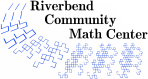# Math Circle Pedagogy

Our Math Circle classes follow the model of the Boston Math Circle program developed by Bob and Ellen Kaplan, now meeting online and known as the Global Math Circle. Our Math Circles have three main pedagogical goals.

Nuts and Bolts
Students will learn and practice math techniques and concepts. This includes notation, terms, computational techniques, and conventions. Students will be exposed to mathematical lore, favorite tricks, and neat true facts.
Wrenches and Hammers
Students will learn about logic, proofs, and the process of doing mathematics. This includes learning how to ask good mathematical questions, how to attack problems, how to deal with being stuck, how to define new terms, and how to use diagrams appropriately.
Workshop Etiquette
Students will learn to interact appropriately with peers in the context of a mathematical investigation. This includes communicating effectively in verbal and written form, balancing personal emotional needs against those of the group, building a collegial atmosphere capable of producing interesting mathematical insights, and enjoying the process of mathematical discovery.
Riverbend Community Math Center
hello@riverbendmath.org
http://riverbendmath.org
(574) 339-9111This work placed into the public domain by the Riverbend Community Math Center.